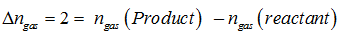# Topic: Equilibrium (Test 1)

Topic: Equilibrium
Q.1
The pH of ammonium acetate will be
A. 7.005
B. 7.0
C. 4.75
D. Between 6 and 7
Explaination / Solution:

is a salt of weak acid and weak base. pH= 7+ 0.5(pKa-pKb) pKa=-log(Ka) and pKb=-log(Kb) Here pKa=pKb so pH=7.
Workspace
Report
Q.2
At a particular temperature and atmospheric pressure, the solid and liquid phases of a pure substance can exist in equilibrium. Which of the following term defines this temperature?
A. Boiling point
B. Normal melting point and Freezing point
C. Equilibrium temperature
D. Phase change temperature
Explaination / Solution:

Normal melting both the phases liquid and solid exist in equilibrium.

Workspace
Report
Q.3
The ionization constant of acetic acid is . Calculate the degree of dissociation of acetic acid in its 0.05 M solution. Calculate the concentration of acetate ion in the solution and its pH.

A. 0.00013M and pH 3.03
B. 0.00093M and pH 3.03
C. 0.00193M and pH 3.03
D. 0.00203M and pH 3.03
Explaination / Solution:

Ka = and pH=-log(Cα).

Workspace
Report
Q.4
Amines behave as
A. Lewis base
B. neutral compound
C. aprotic acid
D. Lewis acids
Explaination / Solution:

Amines behave as lewis base because they have a lp of electrons.

Workspace
Report
Q.5
During the process of transformation from liquid to vapour, the pressure exerted by the water molecules at a given temperature remains constant. This is called
A. melting point of water
B. Boiling point of water
C. freezing point of water
D. vapour pressure of water
Explaination / Solution:

The number of water molecules from the gaseous state into the liquid state also increases till the equilibrium is attained.

i.e.rate of evaporation = rate of condensation
H2O(l) ⇌  H2O (vap)

At equilibrium the pressure exerted by the water molecules at a given temperature remains constant and is called the equilibrium vapour pressure of water increases with temperature.

Workspace
Report
Q.6
The dynamic nature of chemical equilibrium can be demonstrated in the synthesis of ammonia by Haber.s process. Choose the appropriate option given below
A. by using deuterium isotope
B. by using hydrogen isotope
C. by using sulphur isotope
D. by maintaining high temperature and low pressure
Explaination / Solution:

Use of isotope (deuterium) in the formation of ammonia clearly indicates that chemical reactions reach a state of dynamic equilibrium in which the rates of forward and reverse reactions are equal and there is no net change in composition.

Workspace
Report
Q.7
For dissolution of gases in liquids, the concentration of a gas in liquid is.
A. lower to the pressure of the gas as compared to the liquid
B. proportional to the vapour pressure of the gas
C. proportional to the pressure of the gas over the liquid.
D. equal to the pressure of the gas in relation to the liquid
Explaination / Solution:

For dissolution of gases in liquids, the concentration of a gas in liquid is proportional to the pressure of the gas over the liquid

Workspace
Report
Q.8
The value of  for a reaction is directly related to the thermodynamics of the reaction and in particular,
A. Δ G
B. Δngas
C. Qc
D. Qp
Explaination / Solution:

Δ G = -RTlnK

Workspace
Report
Q.9
We know that the relationship between  and is What would be the value of Δngas for the reaction
A. 1
B. 2.0
C. 1.5
D. 0.5
Explaination / Solution:Workspace
Report
Q.10
The pH of neutral water at  is 7.0. As the temperature increases, ionisation of water increases, however, the concentration of  ions and  ions are equal. What will be the pH of pure water at
A. Equal to zero
B. Greater than 7.0
C. Equal to 7.0
D. Less than 7.0